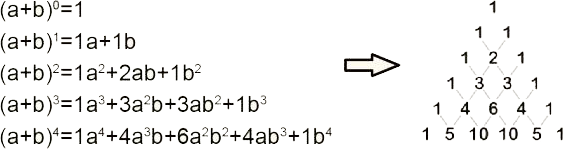## 二项展开式:

$(a+b)^n = C_n^0a^n+C_n^1a^{n-1}b+…+C_n^nb^n$

## 二项展开式系数和三角形的关系如下所示:## 扩展:

 $(a\cdot b)'' = a''+2a'b'+b''$  //系数:1 2 1

Note: 杨辉三角形，是二项式系数在三角形中的一种几何排列，中国南宋数学家杨辉$1261$年所著的《详解九章算法》一书中出现。在欧洲，帕斯卡$1623 \sim 1662$$1654$年发现这一规律，所以这个表又叫做帕斯卡三角形。帕斯卡的发现比杨辉要迟$393$年，比贾宪迟$600$年。
Yang Hui’s Triangle was the same as Pascal’s Triangle